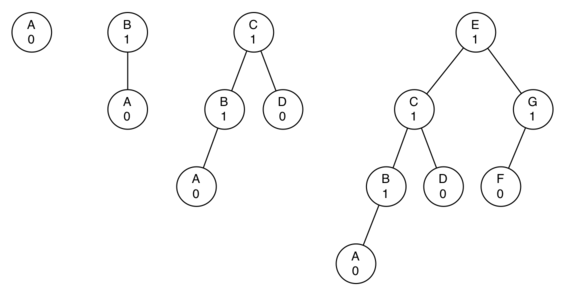# 8.15. AVL Tree Performance¶

Before we proceed any further let’s look at the result of enforcing this new balance factor requirement. Our claim is that by ensuring that a tree always has a balance factor of -1, 0, or 1 we can get better Big-O performance of key operations. Let us start by thinking about how this balance condition changes the worst-case tree. There are two possibilities to consider, a left-heavy tree and a right heavy tree. If we consider trees of heights 0, 1, 2, and 3, Figure 2 illustrates the most unbalanced left-heavy tree possible under the new rules.Figure 2: Worst-Case Left-Heavy AVL Trees

Looking at the total number of nodes in the tree we see that for a tree of height 0 there is 1 node, for a tree of height 1 there is $$1+1 = 2$$ nodes, for a tree of height 2 there are $$1+1+2 = 4$$ and for a tree of height 3 there are $$1 + 2 + 4 = 7$$. More generally the pattern we see for the number of nodes in a tree of height h ($$N_h$$) is:

$N_h = 1 + N_{h-1} + N_{h-2}$

This recurrence may look familiar to you because it is very similar to the Fibonacci sequence. We can use this fact to derive a formula for the height of an AVL tree given the number of nodes in the tree. Recall that for the Fibonacci sequence the $$i_{th}$$ Fibonacci number is given by:

$\begin{split}F_0 = 0 \\ F_1 = 1 \\ F_i = F_{i-1} + F_{i-2} \text{ for all } i \ge 2\end{split}$

An important mathematical result is that as the numbers of the Fibonacci sequence get larger and larger the ratio of $$F_i / F_{i-1}$$ becomes closer and closer to approximating the golden ratio $$\Phi$$ which is defined as $$\Phi = \frac{1 + \sqrt{5}}{2}$$. You can consult a math text if you want to see a derivation of the previous equation. We will simply use this equation to approximate $$F_i$$ as $$F_i = \Phi^i/\sqrt{5}$$. If we make use of this approximation we can rewrite the equation for $$N_h$$ as:

$N_h = F_{h+1} - 1, h \ge 1$

By replacing the Fibonacci reference with its golden ratio approximation we get:

$N_h = \frac{\Phi^{h+2}}{\sqrt{5}} - 1$

If we rearrange the terms, and take the base 2 log of both sides and then solve for $$h$$ we get the following derivation:

$\begin{split}\log{N_h+1} = (h+2)\log{\Phi} - \frac{1}{2} \log{5} \\ h = \frac{\log{N_h+1} - 2 \log{\Phi} + \frac{1}{2} \log{5}}{\log{\Phi}} \\ h = 1.44 \log{N_h}\end{split}$

This derivation shows us that at any time the height of our AVL tree is equal to a constant(1.44) times the log of the number of nodes in the tree. This is great news for searching our AVL tree because it limits the search to $$O(\log{N})$$.

Next Section - 8.16. AVL Tree Implementation